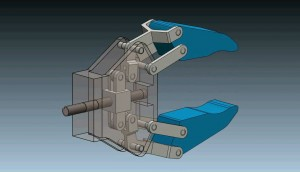# PID Control of Robot Gripper in Matlab / Simulink

In this Tutorial we will see how we to make PID control of 3D Model of a Robot Gripper Mechanism. The Simulink model is organized in two subsystems. A DC Motor subsystem which is model using Simulink blocks and a 3D Model which is imported from Solidworks using the SimMechanics Link. We use a step function to activate the DC Motor to drive the Robot Gripper and we use the Simulink PID Block to automatically tune the PID’s parameters....1.•